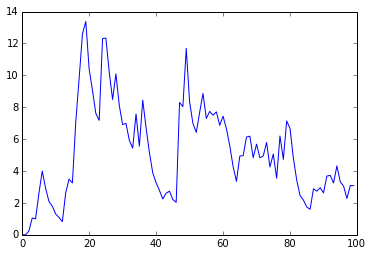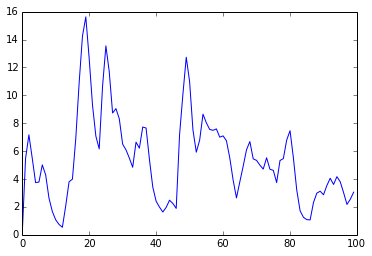### Linear reservoir¶

This is a simple rainfall runoff model. Precipitation is randomly generated from an exponential distribution. Streamflow is governed by $\frac{dS}{dt} = -kS$, where $S$ is the storage in the reservoir.

In :
import sys
sys.path.append('./../')

In :
from stockflow import simulation
import numpy as np
from matplotlib import pyplot as plt


Set up the timestep vector and sample $P$ values. t should contain all the points where you want to evaluate the state variables; it is not necessarily where the ODE solver will sample. More on that in a bit.

In :
tmax = 100
dt = 1
t = np.arange(0,tmax,dt)
P = 5*np.random.standard_exponential(len(t),)


Obviously you'll want to pay attention to units here. This is just an example.

In :
k = 0.3


Now create the simulation object, create stocks with their initial conditions (in a dictionary), and create flows one by one with functions describing their behavior as a function of t.

In :
s = simulation(t)
s.stocks({'S': 0})

# discrete forcing - use discrete=True keyword when calling s.run()
s.flow('P', start=None, end='S', f=lambda t: P[t])
s.flow('Q', start='S', end=None, f=lambda t: k*s.S)
s.run(discrete=True)


The functions can either be lambdas, or defined in the usual way with def my_func(t): .... If you're using discrete forcing data, note that P[t] may be given index values t that are not integers. By default NumPy rounds these down to the nearest integer. This won't matter if your forcing is continuous in t.

Now that the simulation is done, we can access all stock/flow timeseries using s.Q or s.S, for example. Let's plot streamflow.

In :
plt.plot(t, s.Q)
plt.show()Pretty easy. What does the output look like if we change $k$?

In :
k = 0.95
s.run()

In :
plt.plot(t, s.Q)
plt.show()You can see here the response is much more "flashy" with $k=0.95$ than with $k=0.3$ above. If we had the real $Q$ data we could compare them, calculate error, etc.